# Function (mathematics)

In mathematics, a function from a set X to a set Y assigns to each element of X exactly one element of Y. The set X is called the domain of the function and the set Y is called the codomain of the function. The earliest known approach to the notion of function can be traced back to works of Persian mathematicians Al-Biruni and Sharaf al-Din al-Tusi. Functions were originally the idealization of how a varying quantity depends on another quantity. For example, the position of a planet is a function of time. Historically, the concept was elaborated with the infinitesimal calculus at the end of the 17th century, and, until the 19th century, the functions that were considered were differentiable (that is, they had a high degree of regularity). The concept of a function was formalized at the end of the 19th century in terms of set theory, and this greatly enlarged the domains of application of the concept. A function is most often denoted by letters such as f, g and h, and the value of a function f at an element x of its domain is denoted by f(x); the numerical value resulting from the function evaluation at a particular input value is denoted by replacing x with this value; for example, the value of f at x = 4 is denoted by f(4). When the function is not named and is represented by an expression E, the value of the function at, say, x = 4 may be denoted by E|x=4. For example, the value at 4 of the function that maps x to may be denoted by (which results in 25). A function is uniquely represented by the set of all pairs (x, f (x)), called the graph of the function, a popular means of illustrating the function. When the domain and the codomain are sets of real numbers, each such pair may be thought of as the Cartesian coordinates of a point in the plane. Functions are widely used in science, engineering, and in most fields of mathematics. It has been said that functions are "the central objects of investigation" in most fields of mathematics. (Wikipedia).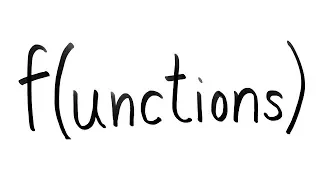What is a function?

This video explains what a mathematical function is and how it defines a relationship between two sets, the domain and the range. It also introduces three important categories of function: injective, surjective and bijective.

From playlist Foundational Math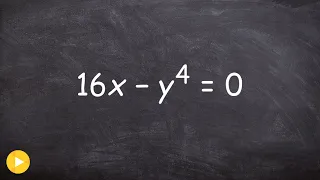Functions of equations - IS IT A FUNCTION

👉 Learn how to determine whether relations such as equations, graphs, ordered pairs, mapping and tables represent a function. A function is defined as a rule which assigns an input to a unique output. Hence, one major requirement of a function is that the function yields one and only one r

From playlist What is the Domain and Range of the Function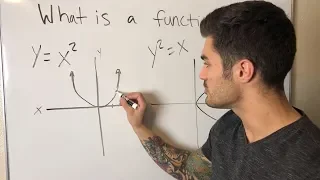What is a Function?

In this video, I talk about the definition of a function and properties of functions. I also go over some examples of how to determine whether a relation is a function or not and how to evaluate functions. Enjoy! Facebook: https://www.facebook.com/braingainzofficial Instagram: https://

From playlist College AlgebraWhat is a Function in Math and Physics? (A more intuitive explanation of Function Definition)

0:00 Introduction 3:35 Examples of functions and why we care 14:55 Make own function 19:12 Definition of a function 21:48 Outro

From playlist Summer of Math Exposition Youtube Videos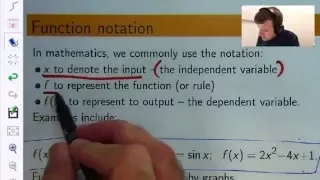What is a Function? Calculus for Beginners: Dr Chris Tisdell Live Stream

What is a function and how are they useful? This video will answer these questions from an elementary mathematics point of view. Functions are a bit like a machine that follows a processing rule. You input something (like a number), the machine processes the number according to the rule,

From playlist Calculus for Beginners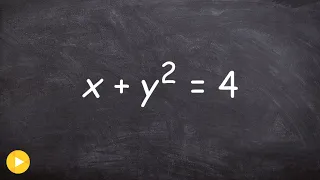Determine if the equation represents a function

👉 Learn how to determine whether relations such as equations, graphs, ordered pairs, mapping and tables represent a function. A function is defined as a rule which assigns an input to a unique output. Hence, one major requirement of a function is that the function yields one and only one r

From playlist What is the Domain and Range of the Function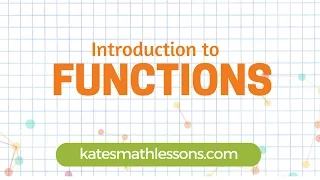What is a Function?

What is a function? How do you use the vertical line test? Learn more about functions and determine if mappings, sets of ordered pairs, tables, or graphs are functions in this short algebra video. Need more math help? Check out our algebra and geometry lessons at katesmathlessons.com

From playlist Algebra 1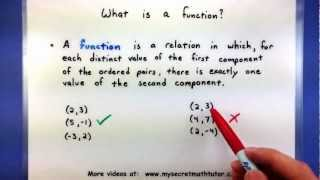Pre-Calculus - Vocabulary of functions

This video describes some of the vocabulary used with functions. Specifically it covers what a function is as well as the basic idea behind its domain and range. For more videos visit http://www.mysecretmathtutor.com

From playlist Pre-Calculus - Functions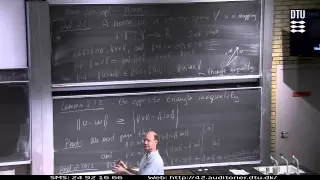Normed Vector Spaces Part 1

Lecture with Ole Christensen. Kapitler: 00:00 - Introduction; 06:45 - Vector Spaces; 07:15 - Example 1; 12:00 - Mathematical Tool - Fourier Transform; 17:00 - Example 2; 20:00 - Example 3; 23:00 - New Concept - Norm; 27:45 - Lemma 2.1.2 - The Opposite Triangle Inequality; 35:15 - Convergen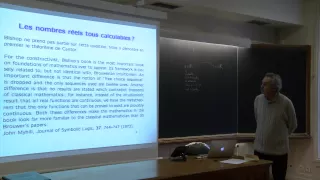SHM - 16/01/15 - Constructivismes en mathématiques - Henri Lombardi

Henri Lombardi (LMB, Université de Franche-Comté), « Foundations of Constructive Analysis, Bishop, 1967 : une refondation des mathématiques, constructive, minimaliste et révolutionnaire »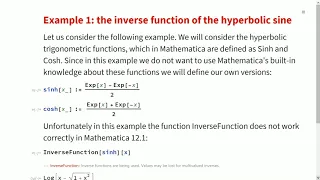Analysis with Mathematica

In this presentation, you'll hear from University of Warsaw professors sharing their experience teaching an analysis course using Mathematica. The presenters give examples of problems where Mathematica can be used effectively as an aid in solving mathematical problems, or at least to inspi

From playlist Wolfram Technology Conference 2020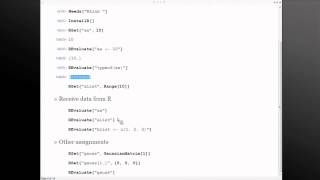Mathematica Experts Live: Integration with R using RLink

Yu-Sung Chang gives an overview of Mathematica's built-in integration with R using RLink as part of Mathematica Experts Live: New in Mathematica 9. For more information about Mathematica, please visit: http://www.wolfram.com/mathematica

From playlist Mathematica Experts Live: New in Mathematica 9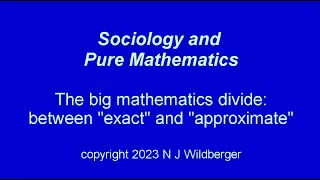The big mathematics divide: between "exact" and "approximate" | Sociology and Pure Maths | NJW

Modern pure mathematics suffers from a major schism that largely goes unacknowledged: that many aspects of the subject are parading as "exact theories" when in fact they are really only "approximate theories". In this sense they can be viewed either as belonging more properly to applied ma

From playlist Sociology and Pure Mathematics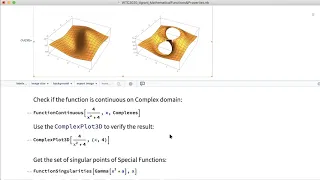Mathematical Functions and Properties

The Wolfram Language has over 250 mathematical functions, including well-known elementary and special functions that have played a crucial role in the development of science for decades. Although this set is almost complete, we are continuously implementing new functionality for mathematic

From playlist Wolfram Technology Conference 2020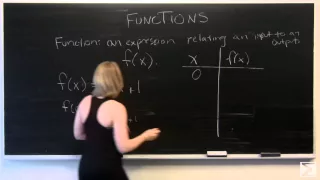Intro to Functions

As part of the college algebra series, this Center of Math video will teach you the basics of functions, including how they're written and what they do.

From playlist Basics: College Algebra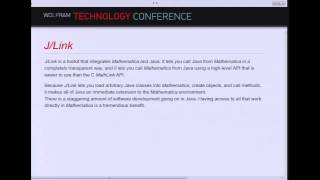Mathematica Connectivity

In this presentation from the Wolfram Technology Conference, Todd Gayley provides an overview of the main connectivity tools in Mathematica and shares guidelines about deciding which one is right for any specific application. For more information about Mathematica, please visit: http://ww

From playlist Wolfram Technology Conference 2012